• 请写出下列表达式，并编写程序验证。


(1) 3.5*3+2*7-'a'
(2) 26/3+34%3+2.5
(3) 45/2+(int)3.14159/2
(4) a=b=(c=a+=6)
设 a
的初值为 3
(5) a=3*5,a=b=3*2
(6) (int)(a+6.5)%2+(a=b=5) 设
a 的初值为
3
(7) x+a%3*(int)(x+y)%2/4 设
x=2.5,a=7,y=4.7(8)(float)(a+b)/2+(int)x%(int)y
设 a=2,b=3,x=3.5,y=2.5

#include <iostream>

using
namespace std;

int main()

{

cout <<  3.5*3+2*7-'a'
<< endl;

cout <<  26/3+34%3+2.5
<< endl;

cout <<  45/2+(int)3.14159/2
<< endl;

int a =
3,b,c;

cout << (a=b=(c=(a+=6))) << endl;

cout<< (a=3*5) <<endl;

cout<< (a=b=3*2) <<endl;

a=3;

cout <<   (int)(a+6.5)%2+(a=b=5)
<< endl;

int x=2.5,y=4.7;a=7;

cout <<  x+a%3*(int)(x+y)%2/4
<< endl;

a=2,b=3,x=3.5,y=2.5;

cout <<  (float)(a+b)/2+(int)x%(int)y
<< endl;

return
0;

}

打印：

-72.5
11.5
23
9
15
6
6
2
3.5


展开全文• 2.6 package booksTest; import seventh.ScannerFileTest; ...import java.util.Scanner;... public static void main(String... 编写程序 ，要求用户从键盘输入a 、b 、和c的，计算下列表达式 */ Scanner sc =
2.6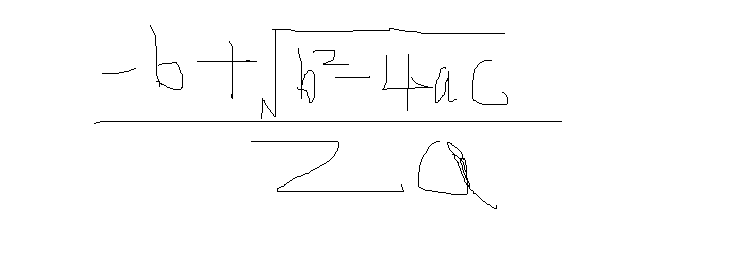package booksTest;

import seventh.ScannerFileTest;

import java.util.Scanner;

public class p34_2_6 {
public static void main(String[] args) {
/*
题目要求
编写程序 ，要求用户从键盘输入a 、b 、和c的值，计算下列表达式的值
*/
Scanner sc = new Scanner(System.in);
for (int i = 0; i <10 ; i++) {
System.out.println("请输入a的值");
double a = sc.nextDouble();
System.out.println("请输入b的值");
double b = sc.nextDouble();
System.out.println("请输入c的值");
double c = sc.nextDouble();
double e = Math.sqrt(b * b - 4 * a * c);
double f = -b + e;
if (e > 0) {
System.out.println("表达式的值为" + f / 2 * a);
return;
} else {
System.out.println("你输入的值b^2-4ac小于0");
}
}
}
}


展开全文• 中缀表达式转后缀表达式：中缀表达式转后缀表达式遵循以下原则： 1.遇到操作数，直接输出； 2.栈为空时，遇到运算符，入栈； 3.遇到左括号，将其入栈； 4.遇到右括号，执行出栈操作，并将出栈的元素输出，...
。中缀表达式转后缀表达式：

中缀表达式转后缀表达式遵循以下原则：

1.遇到操作数，直接输出；
2.栈为空时，遇到运算符，入栈；
3.遇到左括号，将其入栈；
4.遇到右括号，执行出栈操作，并将出栈的元素输出，直到弹出栈的是左括号，左括号不输出；
5.遇到其他运算符’+”-”*”/’时，弹出所有优先级大于或等于该运算符的栈顶元素，然后将该运算符入栈；
6.最终将栈中的元素依次出栈，输出。
经过上面的步骤，得到的输出既是转换得到的后缀表达式。
举例：a+b*c+(d*e+f)g    ———> abc+de*f+g*+

图示上述过程：

因为比较懒，而刚好在网上看到画的还不错的图，所以就直接贴过来了哦。希望作者不要怪罪哦。。。
遇到a，直接输出：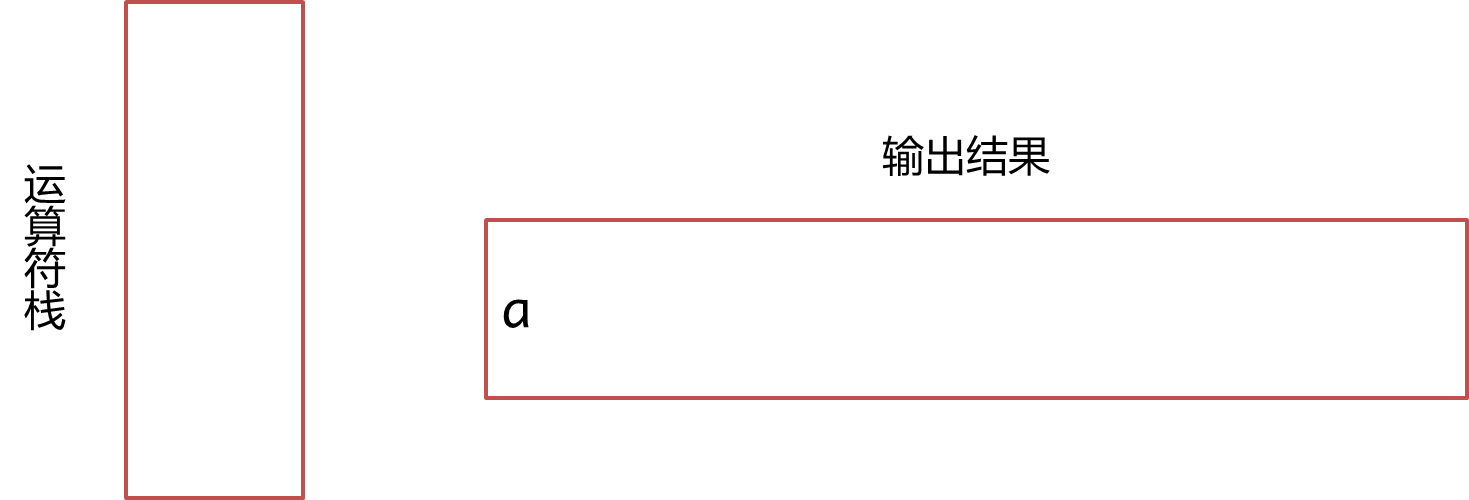遇到+，此时栈为空，入栈：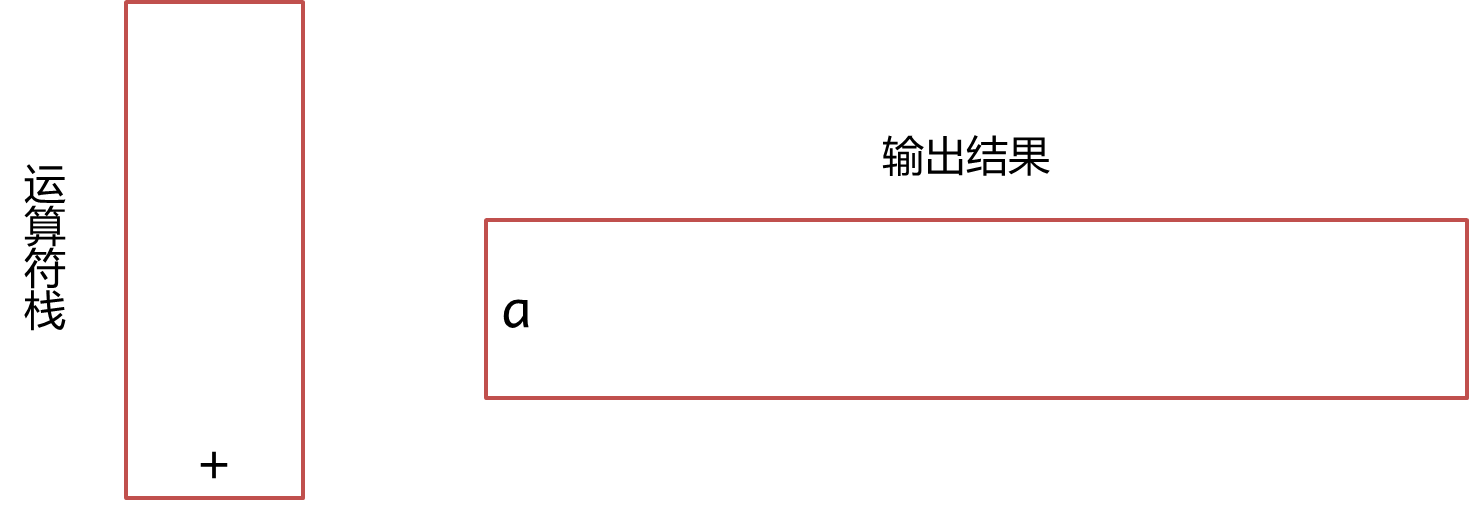遇到b，直接输出：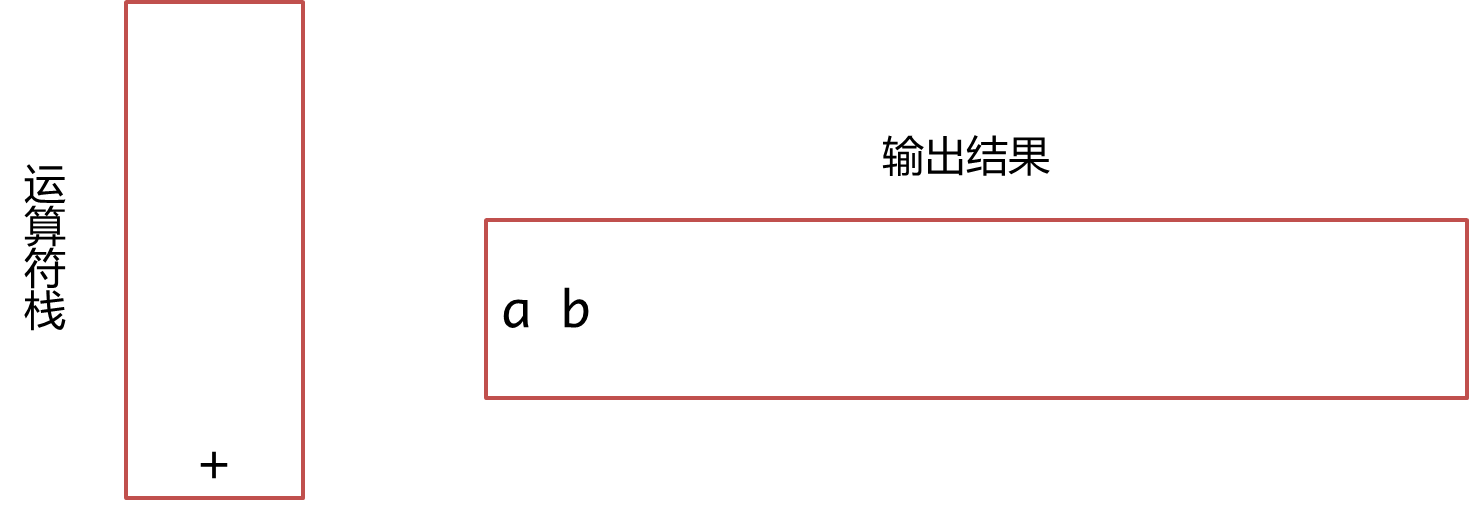遇到*,优先级大于栈顶符号优先级，入栈：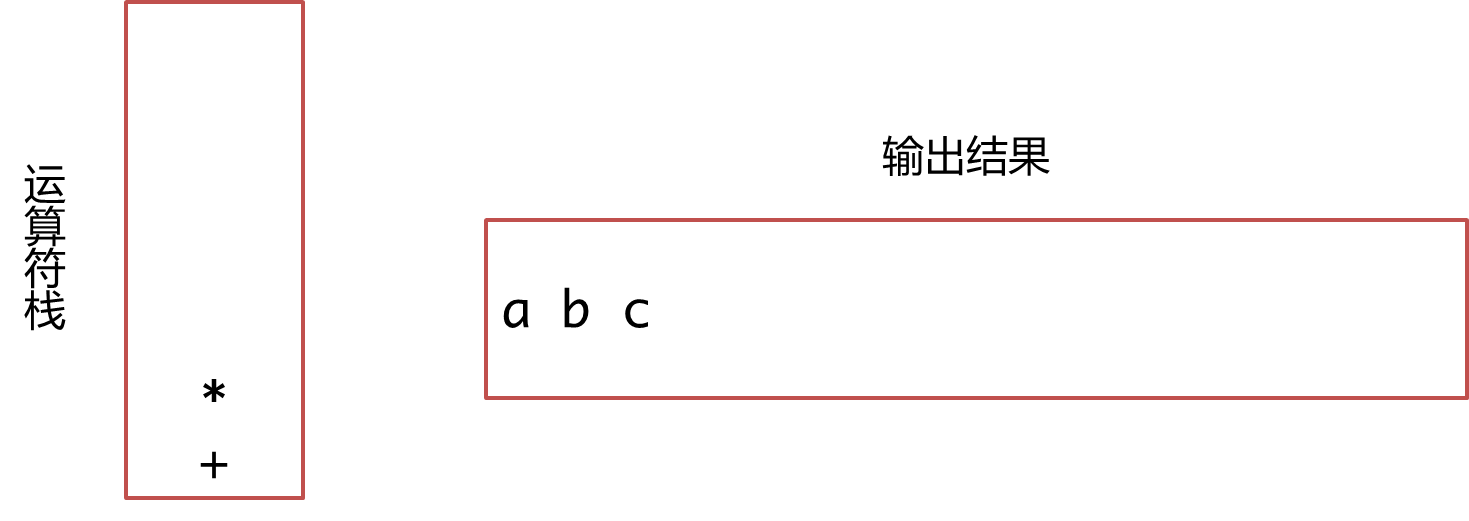遇到c，输出：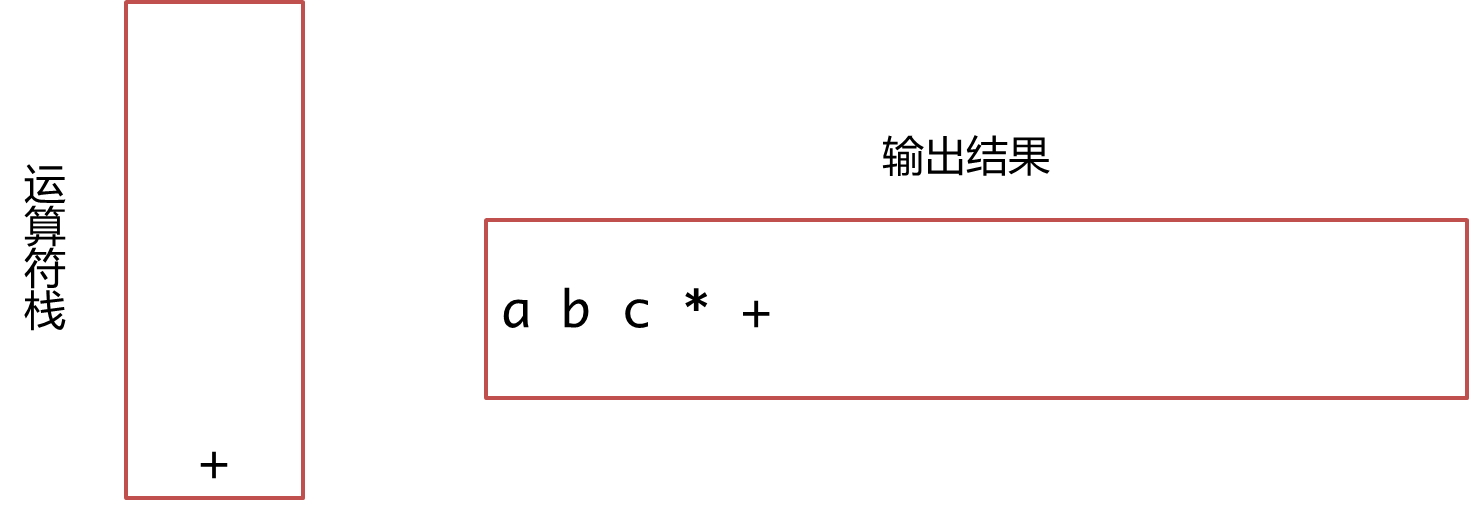到+，目前站内的与+优先级都大于或等于它，因此将栈内的，+依次弹出并且输出，并且将遇到的这个+入栈：遇到(,将其入栈：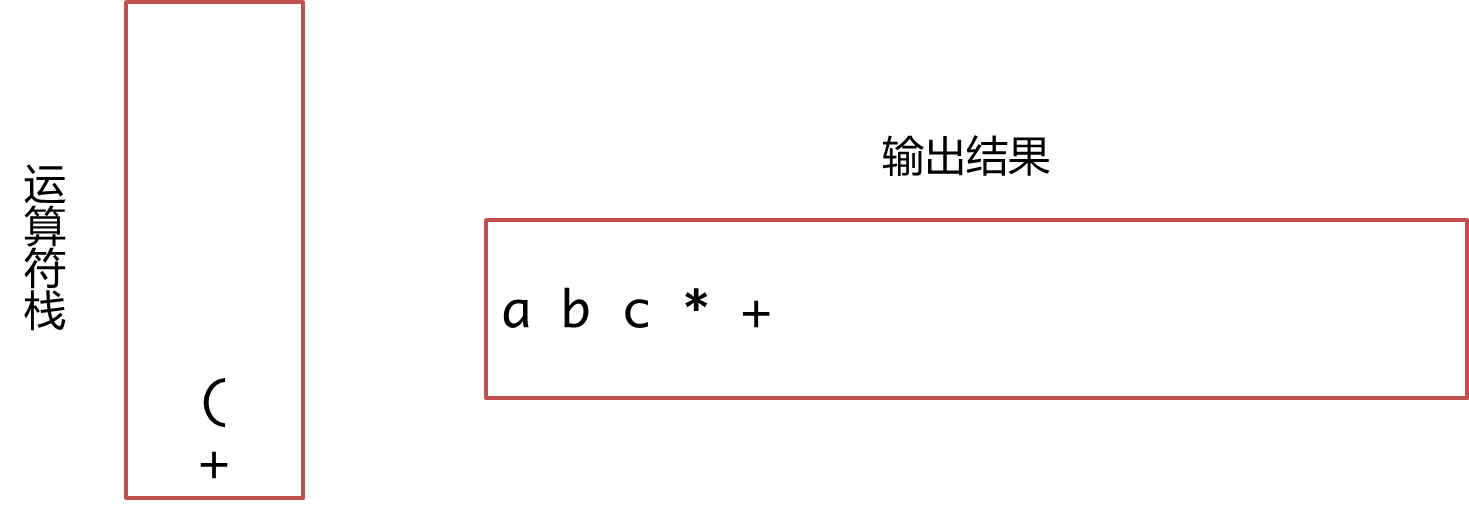遇到d，直接输出：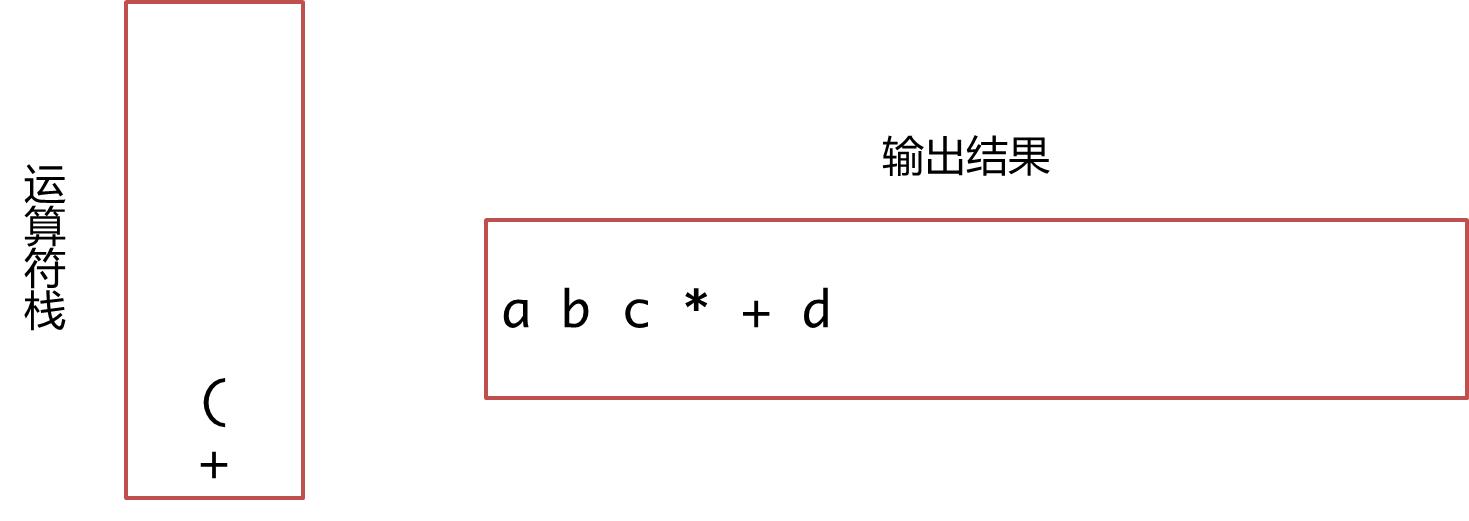遇到*，由于的优先级高于处在栈中的(，因此入栈：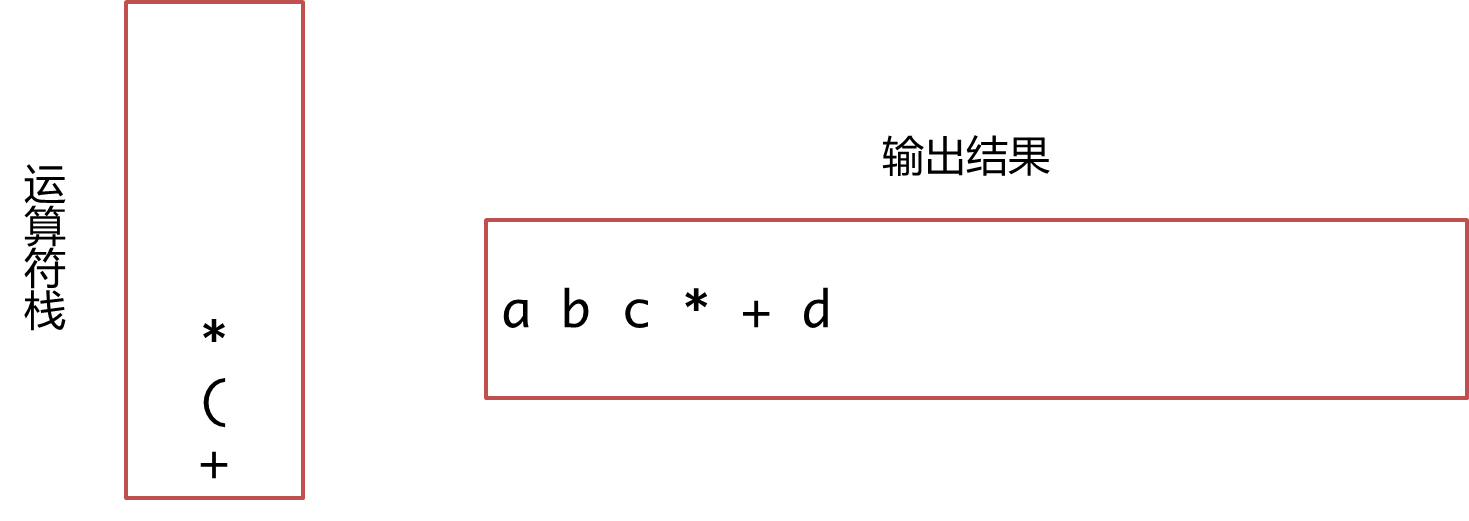遇到e，直接输出：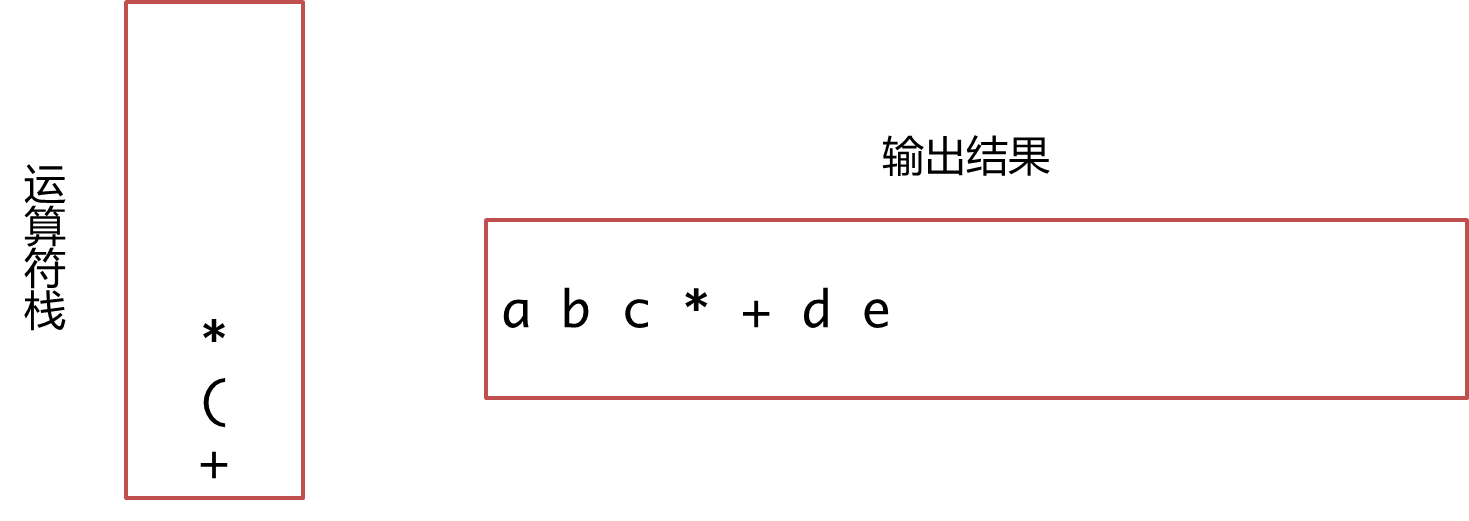遇到+，栈顶的优先级高于+，但是栈内的(低于+，将出栈输出，+入栈：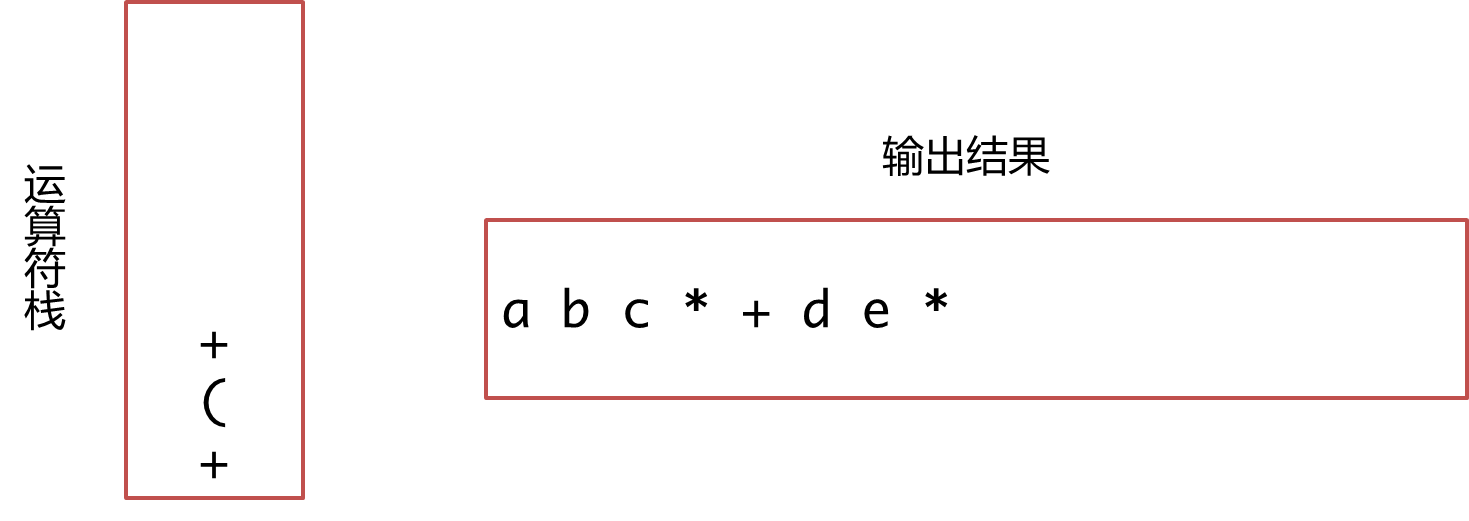遇到f，直接输出：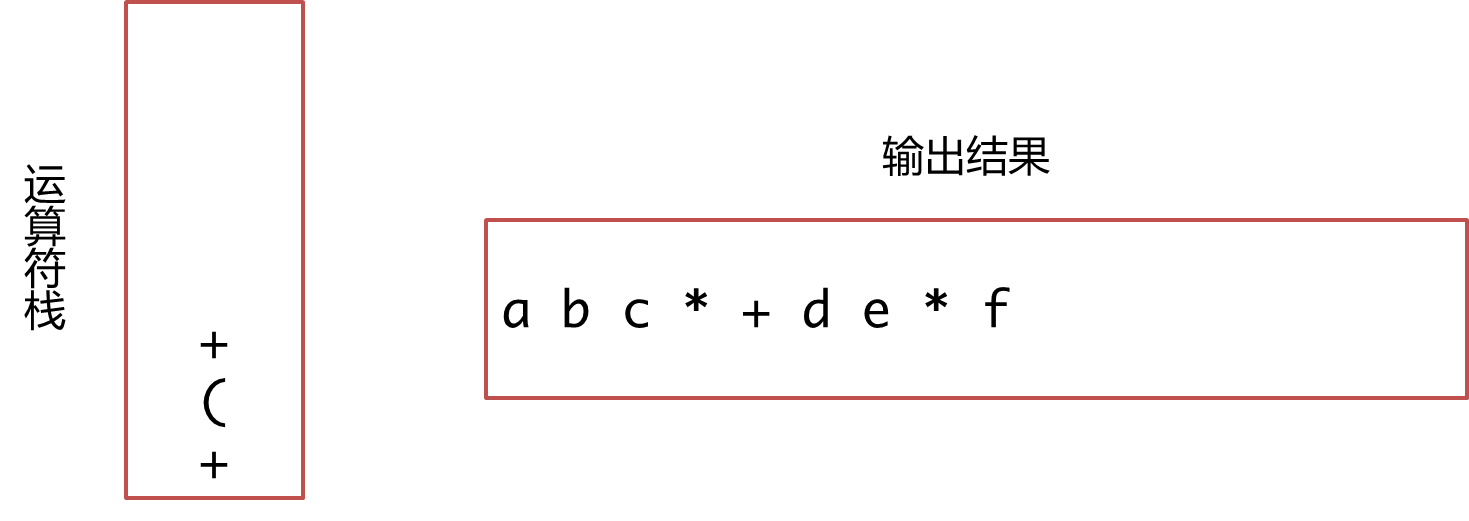遇到),弹出栈顶元素并且输出，直到弹出(才结束，在这里也就是弹出+输出，弹出(不输出：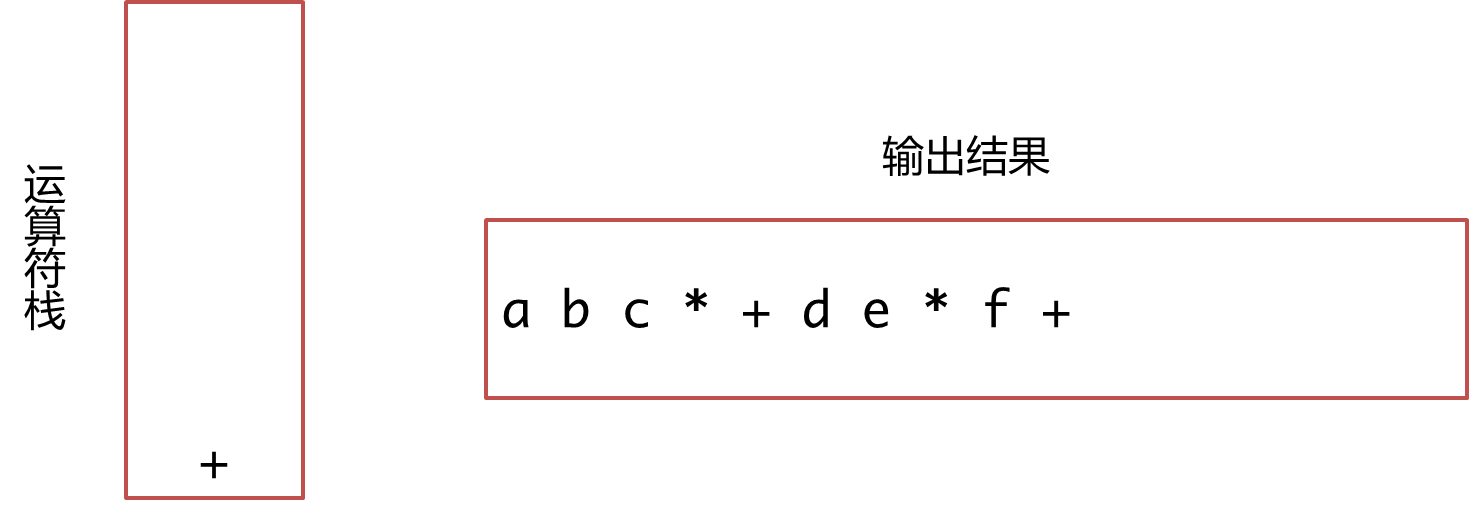遇到*，优先级高于栈顶+，将*入栈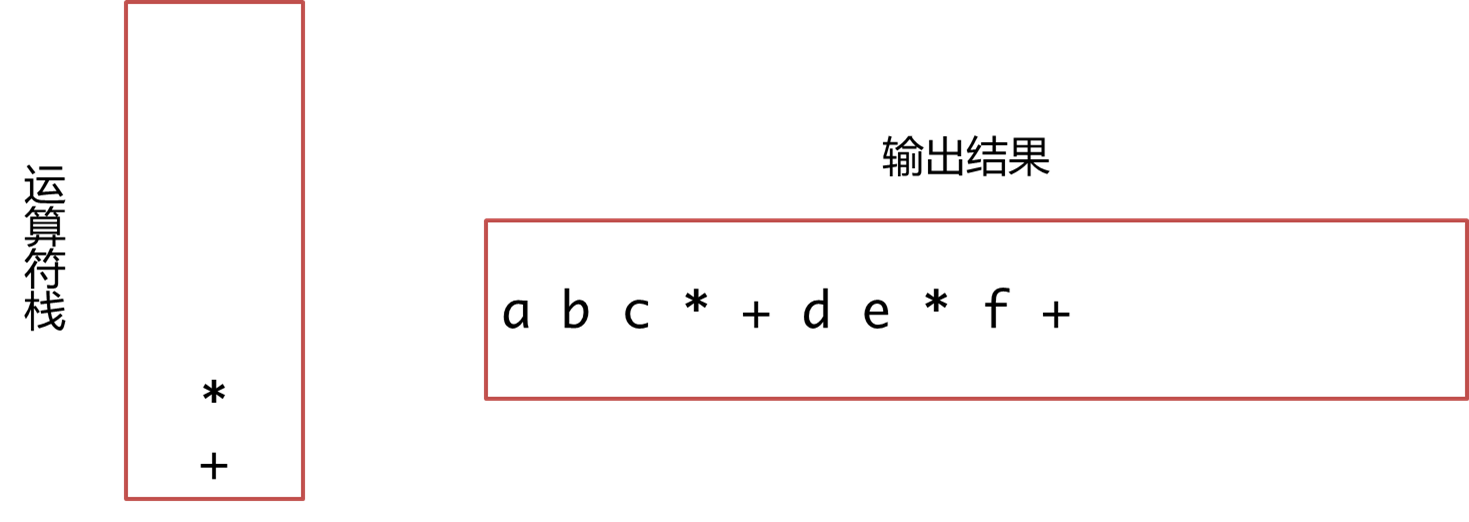遇到g，直接输出： ：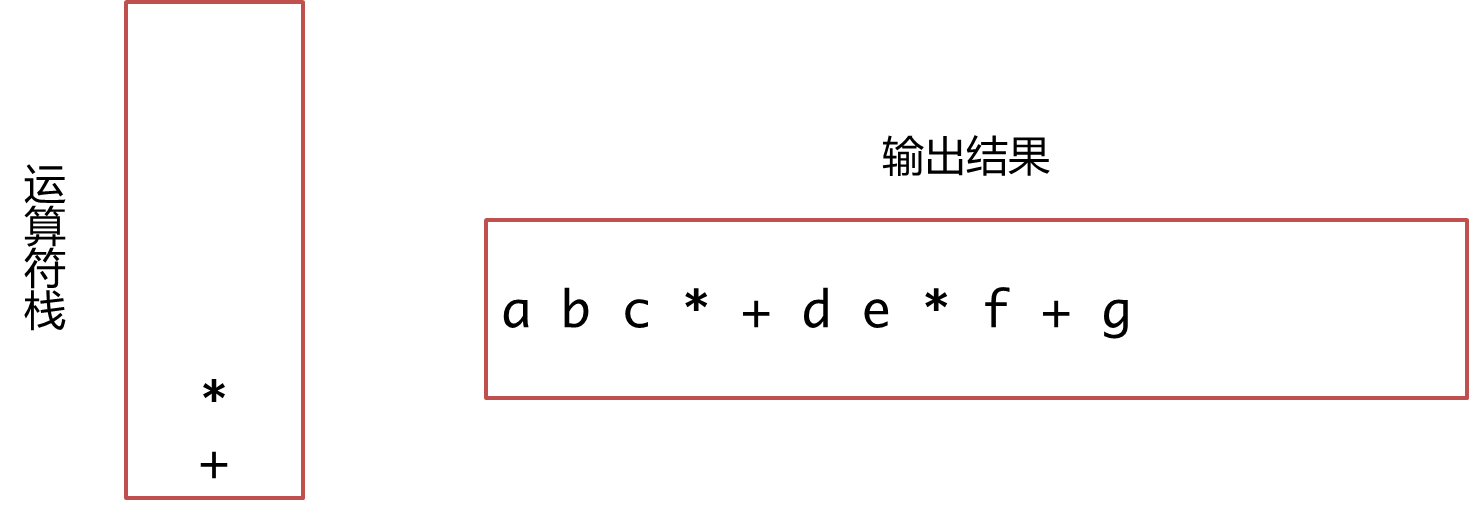此时已经没有新的字符了，依次出栈并输出操作直到栈为空：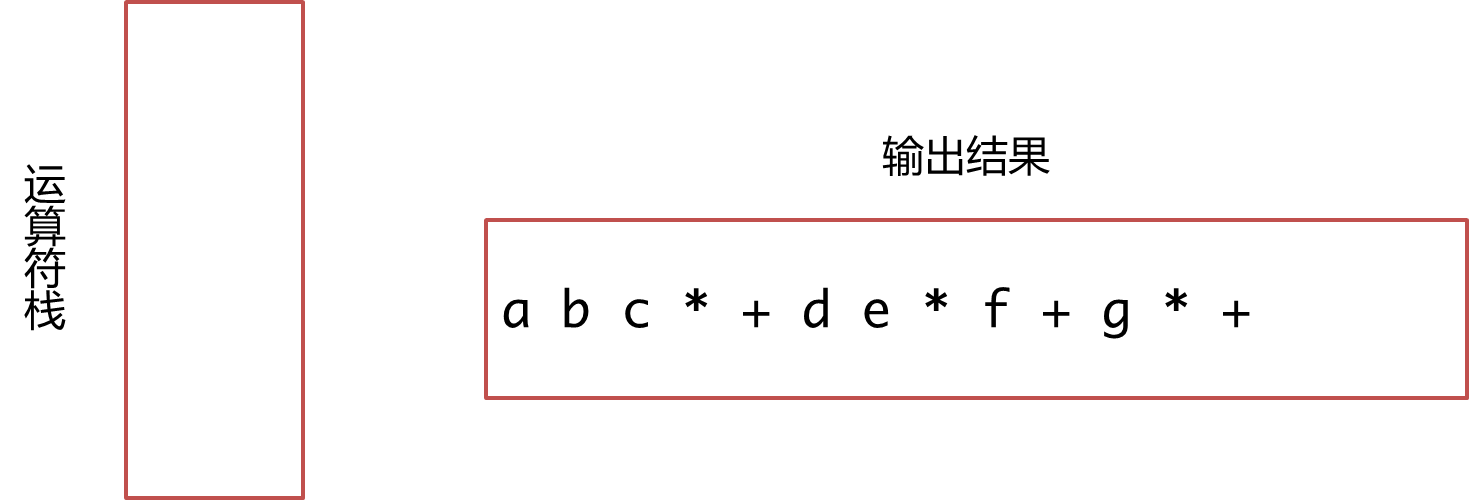因为后缀表达式求值过程较为简单：
所以在这里我只进行简单说明：
1.扫描后缀表达式：
①如果是数字，则让其进栈
②若为操作符，则从栈中取出两个操作数，先取出的作为右操作数，后取出的作为左操作数，然后进行该操作符的运算，并使其结果入栈。
③重复上述过程，直至表达式扫描完成。
2.最终栈中只留一个元素—–>即就是结果。

下面代码实现中缀转后缀以及后缀表达式求值：

使用的栈是自定义栈（自己实现的）：
//stack.h

#define _CRT_SECURE_NO_WARNINGS 1
#include<iostream>
using namespace std;
#include<cassert>

//------------使用类型萃取来拷贝栈内元素-------------
struct _TrueType
{
bool Get()
{
return true;
}
};

struct _FalseType
{
bool Get()
{
return false;
}
};

template<class _Tp>
struct TypeTraits
{
typedef _FalseType _IsPODType;
};

template<>
struct TypeTraits<bool>
{
typedef _TrueType _IsPODType;
};

template<>
struct TypeTraits<int>
{
typedef _TrueType _IsPODType;
};

template<>
struct TypeTraits<unsigned int>
{
typedef _TrueType _IsPODType;
};

template<>
struct TypeTraits<char>
{
typedef _TrueType _IsPODType;
};

template<>
struct TypeTraits< float >
{
typedef _TrueType _IsPODType;
};

template<>
struct TypeTraits< double >
{
typedef _TrueType _IsPODType;
};

template<>
struct TypeTraits<long>
{
typedef _TrueType _IsPODType;
};

template<>
struct TypeTraits< unsigned long>
{
typedef _TrueType _IsPODType;
};

template<class T>
void Copy(T* dst, T* src, size_t size)
{
if (TypeTraits<T>::_IsPODType().Get())
{
memcpy(dst, src, size);
}
else
{
for (int i = 0; i < size; ++i)
{
dst[i] = src[i];
}
}
}

template<class T>
struct TypeTraits< T* >
{
typedef _TrueType _IsPODype;
};

//-------------------------栈的基本操作----------------
template<class T>
class Stack
{
public:
//构造函数
Stack(int capacity = 10)
:_pData(NULL)
, _capacity(capacity)
, _size(0)
{
_pData = new T[capacity];
}

//拷贝构造函数
Stack(const Stack<T>& s)
:_pData(new T[s._capacity])
, _size(s._size)
, _capacity(s._capacity)
{
for (int i = 0; i < _size; ++i)
{
_pData[i] = s._pData[i];
}
}

//赋值运算符函数
Stack& operator=(Stack<T> s)
{
std::swap(_pData, s._pData);
_size = s._size;
_capacity = s._capacity;
return *this;
}

//入栈
void Push(const T& data)
{
CheckCapacity();
_pData[_size++] = data;
}
//出栈
void Pop()
{
if (!Empty())
{
--_size;
}
}

//获取栈顶元素
T& Top()
{
if (!Empty())
{
return _pData[_size - 1];
}
}

const T& Top()const
{
if (!Empty())
{
return _pData[_size - 1];
}
}

size_t Size()const
{
return _size;
}

bool Empty()const
{
return 0 == _size;
}

//析构函数（释放资源）
~Stack()
{
if (_pData)
{
delete[] _pData;
_pData = NULL;
}
}

private:
//增容
void CheckCapacity()
{
if (_size >= _capacity)
{
_capacity = 2 * _capacity + 3;
T* tmp = new T[_capacity];
//拷贝原数据
//释放旧空间
//指向新空间
//需要进行类型萃取
Copy<T>(_pData, tmp, _size);
delete[] _pData;
_pData = tmp;
}
}

T* _pData;
int _capacity;
int _size;
};

//----------------------------------------------------------
//需要用到的函数的声明
int GetPriority(char ch, int flag);//获取优先级
bool IsOperator(char ch);//判断是否为操作符
void prefixionToSuffix(char* dst, char* src);//中缀表达式转后缀表达式
int  SuffixToValue(char *suffix, char *prefixion);//后缀表达式求值



中缀表达式转后缀表达式：
//prefixionToSuffix.cpp

#include"Stack.h"

//flag为1时表示栈内优先级  flag为0表示栈外优先级
int GetPriority(char ch, int flag)
{
if (ch == '+' || ch == '-')
{
if (flag)
{
return 3;
}
else
{
return 2;
}
}
else if (ch == '*' || ch == '/' || ch == '%')
{
if (flag)
{
return 5;
}
else
{
return 4;
}
}
else if (ch == '(')
{
if (flag)
{
return 1;
}
return 6;
}
else if (ch == ')')
{
if (flag)
{
return 6;
}
else
{
return 1;
}
}
}

bool IsOperator(char ch)
{
if (ch == '+' || ch == '-' || ch == '*' || ch == '/' || ch == '%' || ch == '(' || ch == ')')
{
return true;
}
return false;
}

//中缀表达式转后缀表达式
void prefixionToSuffix(char* dst, char* src)
{
assert(dst);
assert(src);
Stack<char> s;
char * cur = src;
char* tmp = dst;
while (*cur != '\0')
{
if (*cur >= '0' && *cur <= '9')
{
*tmp++ = *cur;
cur++;
continue;
}
else if (IsOperator(*cur))
{
if (s.Empty())//如果栈为空，直接入栈
{
s.Push(*cur);
cur++;
}
else//如果栈不空，则需要判断栈顶元素和当前操作符的优先级
{
if (*cur == ')')
{
while (*cur == ')' && s.Top() != '(')
{
*tmp++ = s.Top();
s.Pop();
}
s.Pop();
cur++;
}
if (GetPriority(*cur, 0) > GetPriority(s.Top(), 1))
{
s.Push(*cur);
cur++;
}
else
{
while (!s.Empty() && GetPriority(*cur, 0) < GetPriority(s.Top(), 1))
{
*tmp++ = s.Top();
s.Pop();
}
s.Push(*cur);
cur++;
}

}
}
else
{
*tmp++ = *cur++;
}
}
while(!s.Empty())
{
*tmp++ = s.Top();
s.Pop();
}
}


后缀表达式求值：
//SuffixToValue.cpp

#include"Stack.h"

//12 3 4 + * 6 - 8 2 / +
int  SuffixToValue(char *suffix, char *prefixion)
{
prefixionToSuffix(suffix, prefixion);

Stack<int> s;
char* cur = suffix;
int res = 0;
int tmp = 0;
while (*cur != '\0')
{
if (*cur == '+' || *cur == '-' || *cur == '*' || *cur == '/' || *cur == '%')
{
char ch = *cur;
int right = s.Top();
s.Pop();
int left = s.Top();
s.Pop();
switch (ch)
{
case '+':
s.Push(left + right);
break;
case '-':
s.Push(left - right);
break;
case '*':
s.Push(left * right);
break;
case '%':
s.Push(left % right);
break;
case '/':
if (right)
{
s.Push(left / right);
}
break;
}
cur++;
}
else if (*cur >= '0' && *cur <= '9')
{
res = 0;
while (!isspace(*cur) && *cur >= '0' && *cur <= '9')
{
tmp = *cur - '0';
res = res * 10 + tmp;
cur++;
}
s.Push(res);
//cur++;
}
else
{
cur++;
}
}
if (!s.Empty())
{
res = s.Top();
return res;
}
}


main.cpp

#include"Stack.h"

void Test()
{
char prefixion[] = "12 * (3 + 4) - 6 + 8 / 2 ";//保存前缀表达式
char suffix = {};//保存后缀表达式
int res = SuffixToValue(suffix, prefixion);
cout << res << endl;
}

int main()
{
Test();
return 0;
}

展开全文• 1）递归执行下列步骤即可求：先分别求出左子树和右子树表示的子表达式，最后根据根结点的运算符的要求，计算出表达式的最后结果。 2）二叉树的中序遍历序列与原算术表达式基本相同，但是需要将中序序列加上...
C++利用二叉树求表达式的值
1.内容及要求
将通过二叉链表实现的表达式二叉树进行输出，同时计算出结果。
要求：
1）二叉树建立时，使用先序建立；
2）四个运算符包括：+, -, *, /;
3 ) 在输出时，遇到优先级问题时，相应的括号也要输出。
提示：
1）递归执行下列步骤即可求值：先分别求出左子树和右子树表示的子表达式的值，最后根据根结点的运算符的要求，计算出表达式的最后结果。
2）二叉树的中序遍历序列与原算术表达式基本相同，但是需要将中序序列加上括号，即当根结点运算符优先级高于左子树（或右子树）根结点运算符时，就需要加括号。
例如:
输入	Result
-+3@@2@@-4@@1@@/6@@2@@
3+2(4-1)-6/2=6
3@@+/6@@2@@-3@@1@@
3(6/2+3-1)=15
2.具体代码及解析

必要的头文件

#include <iostream>
#include <stdlib.h>
#include<bits/stdc++.h>
using namespace std;

2.二叉树的结点定义
typedef struct node
{
char data;//当前结点对应的值
struct node *lson, *rson;//左子树指针和右子树指针
} *TreeNode,Node;

3.创建二叉树
void creattree(TreeNode &tree)
{
char ch;
cin>>ch;//键盘输入字符
if(ch=='@')//判断该字符是否为结束标志符
{
tree=NULL;
}
else//不是结束标志符，则运用递归思想创建左子树和右子数
{
tree=new Node;
tree->data=ch;
creattree(tree->lson);
creattree(tree->rson);
}
};

4.中序输出表达式
void inOrder(TreeNode tree)
{
if(tree!=NULL)
{
if ((tree->data == '*' || tree->data == '/') && (tree->lson->data == '+' || tree->lson->data == '-')&&(tree->rson->data == '-' || tree->rson->data == '+'))//左右两边都需要加括号
{
cout << "(";
inOrder(tree->lson);
cout << ")";
cout << tree->data;
cout << "(";
inOrder(tree->rson);
cout << ")";
}
else if ((tree->data == '*' || tree->data == '/') && (tree->lson->data == '+' || tree->lson->data == '-' || tree->rson->data == '-'|| tree->rson->data == '+'))
{
inOrder(tree->lson);
cout << tree->data;
cout << "(";
inOrder(tree->rson);
cout << ")";
}
else
{
inOrder(tree->lson);
cout << tree->data;
inOrder(tree->rson);
}
}
}

5.计算上述表达式的值
int jisuan(TreeNode tree)
{
if(tree)
{
if(tree->data>='0'&&tree->data<='9')
{
return tree->data-'0';
}
else
{
if(tree->data=='+')
{
return jisuan(tree->lson)+jisuan(tree->rson);
}
else if(tree->data=='-')
{
return jisuan(tree->lson)-jisuan(tree->rson);
}
else if(tree->data=='*')
{
return jisuan(tree->lson)*jisuan(tree->rson);
}
else if(tree->data=='/')
{
return jisuan(tree->lson)/jisuan(tree->rson);
}
}
}
return jisuan(tree);
};

6.主函数
int main()
{
TreeNode T;
creattree(T);
inOrder(T);
cout<<'=';
cout<<jisuan(T);
return 0;
}

3.运行结果及说明
程序运行结果如下
*2@@-3@@1@@
2*(3-1)=4
Process returned 0 (0x0)   execution time : 3.739 s
Press any key to continue.

-+3@@*2@@-4@@1@@/6@@2@@
3+2*(4-1)-6/2=6
Process returned 0 (0x0)   execution time : 1.543 s
Press any key to continue.


-+3@@*2@@-4@@1@@/6@@2@@
3+2*(4-1)-6/2=6
Process returned 0 (0x0)   execution time : 1.543 s
Press any key to continue.

本题核心在于递归思想的运用，从创建、输出到计算，均运用了递归的思想，将大问题分解成一个个子问题。
（上述代码测试用例并不是很多，如有错误，望请指正，谢谢！）


展开全文数据结构
• A选项中，1<2返回结果 True， and左右两个表达式都为True，即返回结果为True； B选项中， 在逻辑运算符中，其优先级为not>and>or，即not True为False， False or False返回结果为False； C选项中， not ...练习
• /*编制程序，输入m、n（m≥n≥0）后，计算下列表达式并输出。 要求将计算阶乘的运算编写作函数fact(n)，函数返回值的类型为float*/#include<stdio.h> double fact(int j); int main(void) { int m,n;...
• ## 计算表达式的值

千次阅读 2017-08-20 21:15:51
描述 有些语言中表达式的运算符使用字符串表示，例如用mul代表*，用div代表/，用add代表+...输出为n行，每行是对应表达式。注意，此处要求的所有运算均为整数运算。 样例输入 5 345 mul 122 3945 div 12 321 ad
• 要求：设计一个算法，将一般算术表达式转化为逆波兰表达式，并求逆波兰表达式。 实现思路 获取一个中缀表达式表达式转换为后缀表达式 计算后缀表达式的结果 中缀表达式转换为后缀表达式的几个关键部分 ...
• 后缀表达式,如1+(3+1)*(3-2)后缀表达式是131+32-*+ 解决表达式首先需要根据字符串表达式求出后缀表达式，然后使用后缀表达式和操作数栈实现计算， 计算的大致思想是从后缀表达式中取元素，如果元素是数值则...
• 假设算术表达式只包含”+”、“-”、“*”、“/”、正整数和括号的合法数学表达式，求算术表达式值。 过程是：先将算术表达式转换成后缀表达式(逆波兰式)，然后对该后缀表达式求值。 　1)将算术表达式exp转换成...
• 　中缀表达式的求问题是一个比较常见的问题之一，我们通常在编写程序时，直接写出表达式让编译器去处理，很少去关心编译器是怎么对表达式进行求的，今天我们来一起了解一下其中具体的原理和过程。 　表达式
• Aviator是一个高性能、轻量级的 java 语言实现的表达式引擎, 主要用于各种表达式的动态求。现在已经有很多开源可用的 java 表达式引擎,为什么还需要 Avaitor 呢? Aviator的设计目标是轻量级和高性能,相比...Aviator scala
• #include double fact( int n); int main( void ) { ...1 ...1 ;i;i++ ){ product =product* i; } return product; } 转载于:https://www.cnblogs.com/blgl/p/3379963.html
• 数据结构 表达式 在计算机中，表达式可以有三中不同的标识方法 设 Exp ＝ S1 + OP + S2 则， 称 op + S1 + S2 为表达式的 “前缀表达式” 称 S1 + OP + S2 为表达式的 “中缀表达式” 称 S1 +...
• 源代码 #include<stdio.h>...#define OK 1 #define ERROR 0 #define STACKINCREMENT 5 #define STACK_INIT_SIZE 10 typedef char SElemType; typedef int Status; typedef struct{ SEle...数据结构作业 实习报告
• 中缀表达式就是我们日常生活中使用的习惯性的表达式，例如：1 + 2 * 3，这里我们实现从键盘接受一段中缀表达式，并对其进行求，输出结果。 具体步骤如下： 首先从键盘接受一段中缀表达式。使用Java 栈的应用
• ## 算术表达式求值（C语言栈）

千次阅读 多人点赞 2020-11-16 15:24:08
题目：算术表达式 题目描述：表达式计算是实现程序设计语言的基本问题之一，也是栈的应用的一个典型例子。设计一个程序，演示用运算符优先法对算数表达式的过程。 基本要求：以字符序列的形式从终端输入...算法 数据结构
• 我们知道，在C 语言中经常使用 诸如 if（表达式）{} ，while (表达式) {} 形式的判断语句，当表达式为真时执行 {} 中的函数体，但是当表达式为赋值表达式时怎么判断该表达式为真还是为假？或者当表达式为逗号表达式...
• 算术表达式是数据结构中栈运用的典型例子。一般来说，为了算法的简化和代码可读性方面的考虑，都是把我们所习惯写的中缀表达式转化为后缀表达式后，再进行相关的计算，各种计算器程序的原理也与之类似。 本次...
• Lambda 表达式使用Lambda 运算符 “=>”来定义，语法如下： (参数列表) => {方法体} Lambda 运算符的左边是输入参数，定义Lambda表达式的接收参数列表，右边包含表达式或语句块，表示将表达式或语句块返回的...c# Lambda
•c语言
• 条件表达式为真，程序和打印“x大于5″。逻辑错误可以很容易或很难找到，根据问题的性质。 另一个常见的语义错误是违反假设。一违反假设发生时，程序员的假设的东西都将是真实的或虚假的，而不对。例如： ...
• 给出一个后缀表达式，如 358*2/+6- （中缀表达式为3+5*8/2-6，结果为17），写程序求出它的。 A: 首先想到用栈来解决问题，当读到数字时取出放入栈中，读到运算符时则从栈中取出两个数进行运算并将结果存到栈...java
• 计算下列表达式值： Input 输入x和n的值，其中x为非负实数，n为正整数。 Output 输出f(x,n)，保留2位小数。 Sample Input 3 2 Sample Output 2.00 #include <stdio.h> #include <stdlib.h> double f...
•  表达式的求上，java的选择非常多，强大的如Groovy、JRuby，N年没维护的beanshell，包括javaeye上朋友的IKExpression。为什么还需要Aviator？或者说Aviator的特点是什么？  我将Aviator定位在Groovy这样全...java Aviator
• 假设算术表达式的语义二叉树已经给出，请编码实现算术表达式的中缀形式（保持计算语义）的输出，并计算出该表达式。 要求： 1）使用二叉树的完全前序序列建立表达式的语义二叉树，空子树用符号@表示； 2）算术...数据结构 数据结构与算法实验
• 编写程序实现表达式，即验证某算术表达式的正确性，若正确，则计算该算术表达式。 主要功能描述如下： 1、从键盘上输入表达式。 2、分析该表达式是否合法： （1）是数字，则判断该数字的合法性。若合法，则...
• ## 中缀表达式转换为后缀表达式

千次阅读 多人点赞 2019-07-08 17:31:58
一、为什么要用后缀表达式 将中缀表达式转换成等价的后缀表达式后，求时，不需要再考虑运算符的优先级，只需从左到右扫描一遍后缀表达式即可 二、后缀表达式 后缀表达式也叫做逆波兰表达式，其求过程...算法
• #include<iostream> using namespace std; int main() { double i,n,sum=0; for(i=0;i<=50;i++) { n=2*i+1; sum=sum+1/n; } cout<<sum; return 0; }c++
• 5.10. 复合表达式的求 含有两个或更多操作符的表达式称为复合表达式。 在复合表达式中，操作数和操作符的结合方式决定了整个表达式表达式的结果会因为操作符和操作数的分组结合方式的不同而不同。 操作......